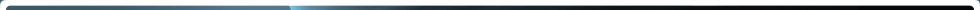Home > NRC Library > Basic References > Glossary > Neutron chain reaction

# Neutron chain reaction

A measure of the intensity of neutron radiation, determined by the rate of flow of neutrons. The neutron flux value is calculated as the neutron density (n) multiplied by neutron velocity (v), where n is the number of neutrons per cubic centimeter (expressed as neutrons/c3) and v is the distance the neutrons travel in 1 second (expressed in centimeters per second, or c/sec). Consequently, neutron flux (nv) is measured in neutrons/cm2-sec.

Page Last Reviewed/Updated Tuesday, June 30, 2020

#### Home